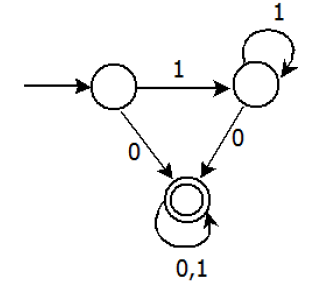# GATE | GATE CS 2013 | Question 33

Consider the DFA given.Which of the following are FALSE?

```1. Complement of L(A) is context-free.
2. L(A) = L((11*0+0)(0 + 1)*0*1*)
3. For the language accepted by A, A is the minimal DFA.
4. A accepts all strings over {0, 1} of length at least 2. ```

(A) 1 and 3 only
(B) 2 and 4 only
(C) 2 and 3 only
(D) 3 and 4 only

Explanation: 1 is true. L(A) is regular, its complement would also be regular. A regular language is also context free.

2 is true.

3 is false, the DFA can be minimized to two states. Where the second state is final state and we reach second state after a 0.

4 is clearly false as the DFA accepts a single 0.

Quiz of this Question

My Personal Notes arrow_drop_up
Article Tags :

Be the First to upvote.

Please write to us at contribute@geeksforgeeks.org to report any issue with the above content.